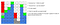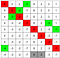# Excuse me, that’s MY SPOT!

Every Jack has his Jill.
Every man can expect to have his partner.

Recently, I did some interesting array-related interview questions. The problem description starts like this or similar: Given an array `nums` containing `n` numbers in the range `[0, n]`and asks you to find duplicate/missing numbers.

Here is one of the problem description

`Given an array of integers where 1 ≤ a[i] ≤ n (n = size of array), some elements appear twice and others appear once.Find all the elements of [1, n] inclusive that do not appear in this array.For example,Input:[4,3,2,7,8,2,3,1]Output:[5,6]`

## The SO-EASY Solution

One can quickly come up with a solution which uses an additional array to store each of the element at its correct spot.

`class Solution {    public List<Integer> findDisappearedNumbers(int[] nums) {        int n = nums.length;        int[] temp = new int[n];        for (var num : nums) {            temp[num - 1] = num;        }        List<Integer> res = new ArrayList<>();        for (int i = 0; i < n; ++i) {            if (temp[i] == 0) {                res.add(i + 1);            }        }        return res;    }}`

That’s it! Thanks for reading ;) I’m kidding. This might be the appetizer or warm-up for the interview and your interviewer can ask you to improve the space or time complexity.

## The Impress Me Solution

One can be asked to improve the space complexity by not using the extra array. Is that achievable? Of course. Recall what is the purpose of the extra array? It’s a temporary place to put each element at its correct spot.

Since each element’s value is within the array’s index, I can actually try to put them in the correct spot by swapping elements of the original array. Here is the illustration.Swap elements to the correct spot. The red block is the current element; the green one indicated the expected spot; the blue ones are elements in the correct spots; the grey ones are extra.

Like the old saying goes: Every Jack has his Jill. We’ve put almost every element in the correct spot except for the extra 2 and 3 in the grey blocks. That’s what the original ask of the interview question. After the swapping, we can iterate the array again and find them.

`public List<Integer> findDisappearedNumbers(int[] nums) {    int n = nums.length;    for (int i = 0; i < n; ++i) {        // swap until nothing to swap        while (nums[nums[i] - 1] != nums[i]) {            int j = nums[i] - 1, temp = nums[i];            nums[i] = nums[j];            nums[j] = temp;        }    }    List<Integer> res = new ArrayList<>();    for (int i = 0; i < n; ++i) {        if (nums[i] != i + 1) {            res.add(i + 1);        }    }    return res;}`

## The Surprise Me Solution

Think about the original problem again, do we need to actually put every element in the correct spot? Well, actually, no. There is another way to claim the spot by flagging. This solution is unique to the problem which uses the property that every element is a positive number. One can flag a spot by flipping the sign of the element in the spot to indicate that there is an element for the spot. Too, verbose, let’s see the illustration. For example, we flipped the sign of 7 to indicate that there is an element, 4 in this case for the spot that 7 sits.The red block is the current element; the green one is the flagging (expected) spot of the current element.

Here is the implementation.

`public List<Integer> findDisappearedNumbers(int[] nums) {    int n = nums.length;    for (int i = 0; i < n; ++i) {        if (nums[Math.abs(nums[i]) - 1] > 0) {            nums[Math.abs(nums[i]) - 1] *= -1;        }    }    List<Integer> res = new ArrayList<>();    for (int i = 0; i < n; ++i) {        if (nums[i] > 0) {            res.add(i + 1);        }    }    return res;}`

## Final Thoughts

Both Impress Me and Surprise Me solutions require modification of the original array to surface the answer and another iteration to extract the answer.

The Impress Me Solution tries to put every element at its correct spot by swapping. I found this approach can be applied to other similar questions even though there might be some better solutions depends on the problem.

Here is another example

`Given an array of integers nums containing n + 1 integers where each integer is in the range [1, n] inclusive.There is only one repeated number in nums, return this repeated number.For example,Input: nums = [1,3,4,2,2]Output: 2`

When comparing the code below with the previous one, they are almost identical.

`public int findDuplicate(int[] nums) {    int n = nums.length;    for (int i = 0; i < n; ++i) {        while (nums[i] != nums[nums[i] - 1]) {            int j = nums[i] - 1, temp = nums[i];            nums[i] = nums[j];            nums[j] = temp;        }    }    for (int i = 0; i < n; ++i) {        if (nums[i] != i + 1) {            return nums[i];        }    }    return -1;}`

Below is another question you can use to practice.

`Given an array nums containing n distinct numbers in the range [0, n], return the only number in the range that is missing from the array.`

The Surprise Me Solution uses in-place flagging to preserve the original information of the array and derive/extract more information. It’s not something new, you’ve probably used it before when solving the famous Game of Life problem where you use additional numbers to flag each cell’s transition or the Set Matrix Zeroes where you use the first column and row to flag whether you need to clear the corresponding rows/columns.

Senior Software Engineer @Hulu

## More from Zengrui Wang

Senior Software Engineer @Hulu